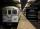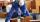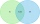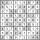# Three integers

Three consecutive integers add up to 51. what are these integers?

a =  16
b =  17
c =  18

### Step-by-step explanation:

$b=a+1=16+1=17$Did you find an error or inaccuracy? Feel free to write us. Thank you!

Showing 1 comment:Asem
This is a cool site and it is going to be very helpful in helping me build my math skills up :). I dropped out of school before I officially turned 16 and always had difficulty with math probably has a lot to do with ADHD. Now I am 26 and going to get my General education degree  and then go back to school :)Tips to related online calculators
Do you have a linear equation or system of equations and looking for its solution? Or do you have a quadratic equation?

## Related math problems and questions:

• Unknown xIf we add to unknown number 21, then divide by 6 and then subtract 51, we get back an unknown number. What is this unknown number?
• My fatherMy father has a big farm. 6/8 of it were planted with mango trees, 1/2 of the remainder are guava trees and the rest 10 trees are santol trees. What is the number of all trees?
• Speed of Slovakian trainsRudolf decided to take the train from the station 'Ostratice' to 'Horné Ozorovce'. In the train timetables found train Os 5409 : km 0 Chynorany 15:17 5 Ostratice 15:23 15:23 8 Rybany 15:27 15:27 10 Dolné Naštice 15:31 15:31 14 Bánovce nad Bebravou 15:35 1
• The temperature 4The temperature was very cold, then it doubled, then it dropped 10 degrees, and then it increased by 40 degrees. The temperature is now 16 degrees. What was the starting temperature?
• Last pageTwo consecutive sheets dropped out of the book. The sum of the numbers on the sides of the dropped sheets is 154. What is the number of the last page of the dropped sheets?
• TrianglesFind out whether given sizes of the angles can be interior angles of a triangle: a) 23°10',84°30',72°20' b) 90°,41°33',48°37' c) 14°51',90°,75°49' d) 58°58',59°59',60°3'
• SubwaySubway train went between two stations that gradually accelerated for 26 seconds and reached a speed of 72 km/h. At this rate, went 56 seconds. Then 16 seconds slowed to a stop. What was the distance between the stations?
• Two-digit numberI am a two-digit number less than 20. When I divided by three, then you get the rest 1 and when you divide me by four you get also rest 1. What number am I?
• Complicated sum minus productWhat must be subtracted from the sum of 3/8 and 5/16 to get difference equal to the product of 5/8 and 3/16?
• SixteenSixteen minus the quotient of 12 and what number is thirteen?
• Odd numbersThe sum of four consecutive odd numbers is 1048. Find those numbers ...
• MonkeyThe monkey fell in 40 meters deep well. Every day it climbs 3 meters, at night it dropped back by 2 m. On what day it gets out from the well?
• BracketsPlace one pair of brackets in expression 20-16 + 10 - 4 - 2 so that the result will be 0.
• Unknown number 24f we add 20, we get 50% of its triple. What is this unknown number?
• Bricklayers8 bricklayers build a house for 630 days. How many bricklayers have to add after 150 days to complete the whole building in (next) 320 days?
• Two setsSuppose Set B contains 69 elements and the total number elements in either Set A or Set B is 124. If the Sets A and B have 29 elements in common, how many elements are contained in set A?
• Even / odd numbersa / Using variable n write two consecutive odd numbers. b / the sum of three consecutive odd numbers is 333. What are this numbers?